## Monday, April 16, 2007

### Aiming a projectile at a moving targetTo hit a moving target by shooting a gun you have to lead it, aiming at the spot where the target will be when the bullet reaches it. In this article we consider three successively harder versions of this problem.

To simplify the math, express the target's position and velocity relative to the shooter's, so that the shooter is effectively stationary at the origin. Assume that the target moves in a straight line, if it is moving. Also assume no air drag.

Motion without gravity

Without gravity, bullets travel in a straight line at constant velocity. To determine where to shoot, imagine shooting an infinite number of bullets simultaneously in all directions, forming an expanding sphere of death. While the sphere is expanding, the target is moving along a straight line (or standing still). If we can determine the instant at which this expanding sphere touches the moving target, we know where to aim: at the target's position when it gets hit by the expanding sphere of death.

Variable definitions:Equation for the time when the target and bullet are equidistant from the shooter:Square both sides:Expand: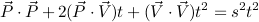Group terms by powers of t:Examine the equation above. If the target's velocity is zero, the impact time works out to simply be target distance divided by bullet speed, which makes sense. The constant term is never negative; it is positive as long as shooter and target are not at the same position. The quadratic term curves upward if the target's speed is faster than the bullet speed. In this case, only the linear term would be capable of bringing the curve below the zero line. In problem terms: if the target is faster than the bullet, the bullet can hit the target only if the target is moving toward the shooter fast enough.

Code for solving this quadratic might look like this:
``double time_of_impact(double px, double py, double vx, double vy, double s){    double a = s * s - (vx * vx + vy * vy);    double b = px * vx + py * vy;    double c = px * px + py * py;    double d = b*b + a*c;    double t = 0;    if (d >= 0)    {        t = (b + sqrt(d)) / a;        if (t < 0)            t = 0;    }    return t;}``
I've done a bit more simplification in going from the equation to the code. Any time there's a 2 on the linear coefficient of the quadratic (which actually happens fairly frequently in my experience), it cancels with the 2 in the denominator and the 4 in the discriminant of the usual quadratic formula. Also, negating the quadratic coefficient (a in the code) cleans out a few minus signs.

Once t is known, plug it into the expression for the motion of the target to find the point to aim at: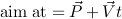This code works equally well with 3D vectors. In a real game I'd be using a vector data type rather than passing around the X, Y, and Z components individually, and I'd have a function for doing dot products instead of writing out x1*x2 + y1*y2 + z1*z2 every time.

If the discriminant (d in the code) is less than zero, it means there are no real roots. In real terms this means the target is moving away faster than the bullet can travel, so there is no possibility of an impact. The code deals with the no-root situation by clamping t to zero, which will cause the gun to aim at the target's current position. A more sensible thing to do might be to find the time t that minimizes distance between bullet and target, and aim at the target's position then.

There is also generally a negative root which corresponds to playing time backwards from the current moment, and as such is not usable.

Space combat games such as Freelancer or Darkstar One draw a targeting reticle ahead of enemy ships, at the spot where you need to shoot in order to hit them. Now you can go forth and do likewise. Obviously, the aim position is different for different bullet speeds, so if you have turbolasers that shoot at 4.5 km/sec and linear tachyon atomizers that shoot at 6 km/sec, you might want two reticles.

Gravity without motion

On earth, gravity pulls bullets downward as they fly. (They also slow down quite a bit due to air resistance, but I'm not going to get into that today.) To counteract gravity you have to aim higher for far-away targets. Let's figure out how to do this with a stationary target first.

Returning to our expanding sphere of death, we could imagine it falling due to gravity as it expands. Equivalently, we could think of the stationary target “falling” upward into the sky instead. This allows us to leave the sphere centered on the origin. Here's the equation for a target which starts at rest and falls upward until it hits the expanding sphere:In a typical game, gravity operates along a single axis. In this case, the G vector will have zeros in all the other components. Squaring: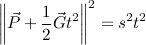Expand:Group terms by power of t:Although we have a fourth power of t, we can substitute u = t2 and be left with a quadratic instead:The two solutions to this u quadratic correspond to the two parabolic arcs that pass through the target point. There's a short, low arc and a longer, high arc. (At maximum range they merge together into one.) Choose whichever one works best for your situation.

Once you've solved for t, plug it into the equation for motion of the target to get the aim position: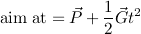Be sure that your targeting code integrates to the same position your simulation will when it moves the bullet through the air. The equations above assume correct integration, but as a kid I wrote things that weren't quite right. For instance:
`newPosition = oldPosition + oldVelocity;newVelocity = oldVelocity + acceleration;`
The code is assuming that velocity is expressed in distance per frame, and acceleration in distance per frame squared. However, it should be adding half the acceleration to the position as well, like this:
`newPosition = oldPosition + oldVelocity + 0.5 * acceleration;newVelocity = oldVelocity + acceleration;`

Gravity and motion together

Finally, let's shoot an arcing projectile at a moving target! The initial equation is just a combination of the starting equations for the previous two cases:Square:Expand: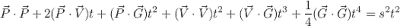Collect terms by power of t:This is a quartic equation and there's no way around it. Let's start by sanity-checking it.

If gravity is zero, the quartic and cubic terms drop out (as well as part of the quadratic term) and we're left with the motion-but-no-gravity equation. If velocity is zero instead, the cubic and linear terms drop out (as well as part of the quadratic term) and we're left with the gravity-but-no-motion equation.

When I'm working on solving problems like this, I find it helpful to rig up my game to plot graphs in real time. In this case, I drive the target around and get a constantly-updated graph of the polynomial and its derivatives for shooting at it. This allows me to quickly get a feel for the nature of the problem. Here's an example graph (with added labels):Looking at the equation, you can see that the fourth-order term will always be positive, which means that the red curve will always turn upward on the outer ends. The constant term will always be positive as long as the target is not at the same place as the shooter, which means the curve will be positive as it crosses the Y axis.

The quartic curve has (up to) three points where it changes direction from upward to downward or vice versa. One way to find the roots would be to divide the curve up into intervals between these points. If one end of an interval is above zero and the other is below zero, you know there is a root within that interval. You could use a binary search to locate it, or you could use the midpoint of the interval as an initial guess and use Newton's method to converge on the root. The outer intervals are unbounded so binary search wouldn't necessarily work there, but you could double a t value until it evaluates positive and then binary search within that interval.

The extrema of the quartic correspond to zeros (roots) of its first derivative (the green line in the graph). The first derivative of a quartic is a cubic. We could follow a similar procedure to find its roots: find the zeros of its derivative (the blue line); use them to subdivide the curve into intervals; evaluate the function at the interval endpoints; and search for roots in the intervals that cross the X axis.

When there are no roots, I decided I wanted my gun to shoot at an angle that brought the bullets as close as possible to the target. This corresponds to finding the non-negative t that gives the smallest value for the quartic function. Minima have to be either at one of the zeros of the first derivative, or at t = 0. Once the roots of the cubic are known it's simple to evaluate the function at them, and at t = 0, and pick the one with the smallest value.

Jochen Schwarze wrote some cubic/quartic solving code in Graphics Gems I which works great.

Once again, once t is known, plug it into the left-hand side of the original equation to get the aim position: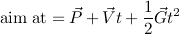Ron Levine wrote a Usenet posting a few years ago covering some of the same ground. There's also a page on the Unreal Wiki.

Joshua Pearce said...

Thank you for posting this online, it was easily the clearest explanation of this concept I could find, and it worked perfectly for my purposes.Anonymous said...

I would really like to thank you for publishing this article, it's been quite helpful to me :)
I don't know how much more time I would have wasted with this problem if it wasn't for you :P

Roman Hwang said...

Thanks. I enjoyed reading the post.

I didn't clearly understand the usage of only one root in Motion without gravity part. You mentioned:

There is also generally a negative root which corresponds to playing time backwards from the current moment, and as such is not usable.

However, isn't a second root b - sqrt(d) can be bigger than zero and smaller than first root.

In suppose it gives better solution, as time to impact will be smaller.

It seems to me, like case when target is moving in direction of shooter. So the expanding sphere (not actually sphere) of all projectiles will have 2 intersections, with one entering and the other quitting.

I suspect that code above will give "quitting", which is still correct, but looks weird - it's much better to shoot in face and early, than later in back.

Please correct me if I'm wrong, I'm just studying such topics and still trying to build solid picture in my head.

James McNeill said...

Roman, I believe you are correct. Good catch!

I'm pretty sure there can only be two positive-time roots if the target is moving more quickly than the projectile, though. Otherwise, once the target's inside the sphere it will always be inside the sphere.

Simran Kaur Khurana said...

Nicely explained tutorial. I wrote a code a object in projectile motion that always hits a moving target.

Hope this helps:
http://lexiconhub.tk/index/game-programming/projectile-motion-unity-3d/

Sven said...

Thanks for this article but even if its quite old I have a problem. I implemented the code but it doesn't hit always. Especially often fails if the target is falling.

I think you can't just add the gravity with the time given by the motion without gravity. Adding the gravity to the position will vary the distance to the target and the time will be incorrect thus the projectile misses.
At least I'm missing a falling target.

I'm not as good in math so i could be completely incorrect but after a few hundreds of shots the minority hits. I would now try to approximate the positions by just running it multiple times and make it getting closer but it uses more cpu than i think must be used.

However predicting a non-falling one works perfect.

James McNeill said...

Sven,

Part of the problem may be in how gravity is getting integrated over time when the projectile moves, versus how the aiming math assumes it will move.

Does your projectile update look something like this?

newVelocity = oldVelocity + gravity * stepDuration
newPosition = oldPosition + oldVelocity * stepDuration

This is an Euler integration step, and it will consistently add too little velocity to the projectile.

A trapezoid integration rule will be much closer to the correct value:

newVelocity = oldVelocity + gravity * stepDuration
newPosition = oldPosition + (oldVelocity + newVelocity) * (stepDuration / 2)

If you are stuck with an Euler step then yeah, the prediction may be off by enough to be a problem. Since gravity is a constant acceleration in a particular direction you might be able to compensate by using a lower gravity constant for the prediction.

James McNeill said...

Sven,

I just thought of something else. You say the target is falling, right? In this case both target and projectile are experiencing the same acceleration, so you should be able to leave gravity out of the prediction entirely.

Does that sound like it could be what is going on?

Sven said...

I can't control the projectile. I just know the velocity and figured the formula of how much I have to aim above the target to hit from a distance.

It's (Distance/ProjVel)^2 * 15 which doesn't seem to be the same gravity than the player at all which is -600.

I let the code draw a path from the predicted points and the player follows it nicely(with targets also having horizontal movement while falling). Even implemented the code from the unreal wiki by Ron Levine just to see that both plot the same path except yours is multiple times faster.

Now I blocked the targets horizontal movement to focus on the prediction but the projectile always flies too high. The distance seems to be proportional to the targets speed somehow.

Unknown said...

First off, lots of great information here!

I've seen some linear intercept functions (no gravity) before, and there are often two positive solutions resulting from the equations, and some care is taken to find the lowest positive intercept time. Yours seem to avoid this sorting. How?

Second, in the final scenario (moving target with gravity), you seem to go off on a sidebar about iterating to find the cubic roots to get the projectile time of flight.
I had wolfram alpha solve the equation for t.
http://www.wolframalpha.com/input/?i=%281%2F4%29%28G+G%29t^4+%2B+%28V+G%29t^3+%2B+%28P+G+%2B+V+V+-+s^2%29t^2+%2B+2%28P+V%29t+%2B+P+P+%3D+0+for+t
This avoids all the iteration, yes? Or have I misunderstood something?

James McNeill said...

Hi Marco,

To answer your first question: Yes, you do have to examine the roots and pick the smallest positive one, if you want shortest time of flight.

Regarding the second question: The solution to a general quartic is a bit involved. (See for instance <a href="http://mathworld.wolfram.com/QuarticEquation.html>this</a>.) Try putting this in Wolfram Alpha:

<pre>a*t^4 + b*t^3 + c*t^2 + d*t + e = 0</pre>

I think the version you fed into Wolfram Alpha treats dot products as if they were scalar products, so it happens to simplify down more. The dot product of P and V, say, is (P_x * V_x + P_y * V_y) in a 2D space; this isn't the same thing as the product of the magnitudes of P and V (unless they happen to be colinear).

I'm not a math genius; I tend to plod through a ton of algebra to get where I'm going. It's certainly possible that the nature of this problem produces something simpler than a general quartic. (My current programming project involves fitting a cubic trajectory to given initial and final positions and velocities, with limits on maximum acceleration, right now; it turns out to be a similar sort of quartic to this trajectory problem.)

Unknown said...

Well this explains why my shots are missing.

I originally was using these formulas to solve ballistics with regard to a stationary target:
Trajectory
And then used this to find flight time:
Time of flight

But I'm having trouble adapting this to hit a moving target.
I don't know if these approaches will lead to a non-iterative solution, or if your approaches might be simply another way of looking at the same thing.

James McNeill said...

The formulas that you mentioned should be equivalent to the gravity-with-no-motion section. (In my explanation, taking the arc-tangent of the aim point to get the launch angle is implied, in part because if you are working in 3D then you would need to do more work to get pitch and yaw angles, but the math of getting a point to aim at is the same.) Computing time of flight from the launch angle, launch speed, and height change seems tricky, though; are they talking about the time when the projectile is at that height going up, or the time when it's at that height coming back down?

I don't think you should be too afraid of doing an iterative solution. It's really easy to come up with problems that are too difficult to solve analytically; for instance, if you add wind drag to your projectile. The math of doing iteration is pretty simple; it's just derivatives of polynomials.

The important thing with Newton iteration is starting at a point that slopes toward the root you're after. That's kind of what I was driving at in the article. You are trying to find the points where a function is zero. If you can find the points where its first derivative is zero, which is a slightly simpler problem, then you can evaluate the function at those points. If the function is positive at one and negative at another, then there must be a zero somewhere in between.

That said, if you are just looking for some drop-in code that you can use, search around the internet. It looks like the link in my post is dead now, but here's another that might serve (I have not tried it out): http://math.ivanovo.ac.ru/dalgebra/Khashin/poly/index.html

Unknown said...

Oh. You're right. That time of flight formula is only for the downward arc.
I found a different one that correctly produces two solutions.
Time of flight
This only leaves this issue of which one to use.
I believe I can simply compare the target location to 1/2 the projectile's max range. If it's greater, use the longer time. If lesser, use the shorter time. Ignore negative times.

I'm currently getting pretty good results with a single aim iteration:
1) Find aim angle to hit target as if stationary.
2) Use flight time based on that angle to predict where target will be.
3) Recalculate aim angle based on predicted location.

Whether or not performance takes a greater hit for finding flight time and recalculating the aim angle, as opposed to Newton iteration is another story.

Thanks for the insight.

schnellbap said...

Hi,

first of all thanks for this nice tutorial!
One (probably) question: Can you please explain this:

"Any time there's a 2 on the linear coefficient of the quadratic (which actually happens fairly frequently in my experience), it cancels with the 2 in the denominator and the 4 in the discriminant of the usual quadratic formula."

I don't see how it cancels the 4 out from the discriminant?

James McNeill said...

The discriminant is sqrt(b^2 - 4*a*c). Let's say b = 2*d (b has a 2 in it, in other words); then we have sqrt((2*d)^2 - 4*a*c), which is sqrt(4*d^2 - 4*a*c), which is 2*sqrt(d^2 - a*c), at which point all terms in the numerator and denominator of the formula are multiplied by 2.

Fishrock123 said...

Thank you for posting this, useful still even 13 years later!

James McNeill said...

You're welcome! I'm glad it is useful.